# Resources tagged with: Properties of numbers

Filter by: Content type:
Age range:
Challenge level:

There are 89 NRICH Mathematical resources connected to Properties of numbers, you may find related items under Numbers and the Number System.

Broad Topics > Numbers and the Number System > Properties of numbers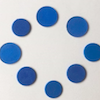### Eightness of Eight

##### Age 5 to 7 Challenge Level:

What do you see as you watch this video? Can you create a similar video for the number 12?### Always, Sometimes or Never? Number

##### Age 7 to 11 Challenge Level:

Are these statements always true, sometimes true or never true?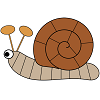### Snail One Hundred

##### Age 5 to 7 Challenge Level:

This is a game in which your counters move in a spiral round the snail's shell. It is about understanding tens and units.### Three Neighbours

##### Age 7 to 11 Challenge Level:

Look at three 'next door neighbours' amongst the counting numbers. Add them together. What do you notice?### Odd Times Even

##### Age 5 to 7 Challenge Level:

This problem looks at how one example of your choice can show something about the general structure of multiplication.### Two Numbers Under the Microscope

##### Age 5 to 7 Challenge Level:

This investigates one particular property of number by looking closely at an example of adding two odd numbers together.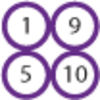### Pairs of Numbers

##### Age 5 to 7 Challenge Level:

If you have ten counters numbered 1 to 10, how many can you put into pairs that add to 10? Which ones do you have to leave out? Why?### Light the Lights Again

##### Age 7 to 11 Challenge Level:

Each light in this interactivity turns on according to a rule. What happens when you enter different numbers? Can you find the smallest number that lights up all four lights?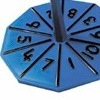### Our Numbers

##### Age 5 to 7 Challenge Level:

These spinners will give you the tens and unit digits of a number. Can you choose sets of numbers to collect so that you spin six numbers belonging to your sets in as few spins as possible?### I Like ...

##### Age 5 to 7 Challenge Level:

Mr Gilderdale is playing a game with his class. What rule might he have chosen? How would you test your idea?### Table Patterns Go Wild!

##### Age 7 to 11 Challenge Level:

Nearly all of us have made table patterns on hundred squares, that is 10 by 10 grids. This problem looks at the patterns on differently sized square grids.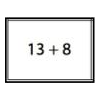### Sort Them Out (1)

##### Age 5 to 7 Challenge Level:

Can you each work out the number on your card? What do you notice? How could you sort the cards?### Elevenses

##### Age 11 to 14 Challenge Level:

How many pairs of numbers can you find that add up to a multiple of 11? Do you notice anything interesting about your results?### Pair Products

##### Age 14 to 16 Challenge Level:

Choose four consecutive whole numbers. Multiply the first and last numbers together. Multiply the middle pair together. What do you notice?### Special Numbers

##### Age 11 to 14 Challenge Level:

My two digit number is special because adding the sum of its digits to the product of its digits gives me my original number. What could my number be?### Cinema Problem

##### Age 11 to 14 Challenge Level:

A cinema has 100 seats. Show how it is possible to sell exactly 100 tickets and take exactly £100 if the prices are £10 for adults, 50p for pensioners and 10p for children.### Sept 03

##### Age 11 to 14 Challenge Level:

What is the last digit of the number 1 / 5^903 ?### Six Times Five

##### Age 11 to 14 Challenge Level:

How many six digit numbers are there which DO NOT contain a 5?### One to Eight

##### Age 11 to 14 Challenge Level:

Complete the following expressions so that each one gives a four digit number as the product of two two digit numbers and uses the digits 1 to 8 once and only once.### Odd Differences

##### Age 14 to 16 Challenge Level:

The diagram illustrates the formula: 1 + 3 + 5 + ... + (2n - 1) = n² Use the diagram to show that any odd number is the difference of two squares.### Summing Consecutive Numbers

##### Age 11 to 14 Challenge Level:

15 = 7 + 8 and 10 = 1 + 2 + 3 + 4. Can you say which numbers can be expressed as the sum of two or more consecutive integers?### Counting Factors

##### Age 11 to 14 Challenge Level:

Is there an efficient way to work out how many factors a large number has?##### Age 7 to 14 Challenge Level:

I added together some of my neighbours' house numbers. Can you explain the patterns I noticed?### Magic Crosses

##### Age 7 to 14 Challenge Level:

Can you find examples of magic crosses? Can you find all the possibilities?### Satisfying Statements

##### Age 11 to 14 Challenge Level:

Can you find any two-digit numbers that satisfy all of these statements?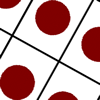### Ten-ness of Ten

##### Age 5 to 7

These tasks will help children understand the 'ten-ness' of ten, a fundamental part of place value.### Guess the Dominoes for Two

##### Age 7 to 14 Challenge Level:

Guess the Dominoes for child and adult. Work out which domino your partner has chosen by asking good questions.### An Introduction to Irrational Numbers

##### Age 14 to 18

Tim Rowland introduces irrational numbers### Take One Example

##### Age 5 to 11

This article introduces the idea of generic proof for younger children and illustrates how one example can offer a proof of a general result through unpacking its underlying structure.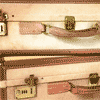### Unlocking the Case

##### Age 7 to 11 Challenge Level:

A case is found with a combination lock. There is one clue about the number needed to open the case. Can you find the number and open the case?### Magic Letters

##### Age 11 to 14 Challenge Level:

Charlie has made a Magic V. Can you use his example to make some more? And how about Magic Ls, Ns and Ws?### Filling the Gaps

##### Age 14 to 16 Challenge Level:

Which numbers can we write as a sum of square numbers?### Few and Far Between?

##### Age 16 to 18 Challenge Level:

Can you find some Pythagorean Triples where the two smaller numbers differ by 1?### Escape from the Castle

##### Age 7 to 11 Challenge Level:

Skippy and Anna are locked in a room in a large castle. The key to that room, and all the other rooms, is a number. The numbers are locked away in a problem. Can you help them to get out?### Generating Triples

##### Age 14 to 16 Challenge Level:

Sets of integers like 3, 4, 5 are called Pythagorean Triples, because they could be the lengths of the sides of a right-angled triangle. Can you find any more?### Difference Dynamics

##### Age 14 to 18 Challenge Level:

Take three whole numbers. The differences between them give you three new numbers. Find the differences between the new numbers and keep repeating this. What happens?##### Age 14 to 16 Challenge Level:

Robert noticed some interesting patterns when he highlighted square numbers in a spreadsheet. Can you prove that the patterns will continue?### A Little Light Thinking

##### Age 14 to 16 Challenge Level:

Here is a machine with four coloured lights. Can you make two lights switch on at once? Three lights? All four lights?### Four Coloured Lights

##### Age 11 to 14 Challenge Level:

Imagine a machine with four coloured lights which respond to different rules. Can you find the smallest possible number which will make all four colours light up?### Guess the Dominoes

##### Age 7 to 14 Challenge Level:

This task depends on learners sharing reasoning, listening to opinions, reflecting and pulling ideas together.### Which Numbers? (2)

##### Age 7 to 11 Challenge Level:

I am thinking of three sets of numbers less than 101. Can you find all the numbers in each set from these clues?### Which Numbers? (1)

##### Age 7 to 11 Challenge Level:

I am thinking of three sets of numbers less than 101. They are the red set, the green set and the blue set. Can you find all the numbers in the sets from these clues?### Sort Them Out (2)

##### Age 7 to 11 Challenge Level:

Can you each work out the number on your card? What do you notice? How could you sort the cards?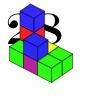### 28 and It's Upward and Onward

##### Age 7 to 11 Challenge Level:

Can you find ways of joining cubes together so that 28 faces are visible?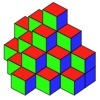### So It's 28

##### Age 5 to 7 Challenge Level:

Here is your chance to investigate the number 28 using shapes, cubes ... in fact anything at all.### Babylon Numbers

##### Age 11 to 18 Challenge Level:

Can you make a hypothesis to explain these ancient numbers?### A Long Time at the Till

##### Age 14 to 18 Challenge Level:

Try to solve this very difficult problem and then study our two suggested solutions. How would you use your knowledge to try to solve variants on the original problem?### What Are Numbers?

##### Age 7 to 18

Ranging from kindergarten mathematics to the fringe of research this informal article paints the big picture of number in a non technical way suitable for primary teachers and older students.### Numbers Numbers Everywhere!

##### Age 5 to 11

Bernard Bagnall recommends some primary school problems which use numbers from the environment around us, from clocks to house numbers.